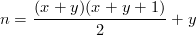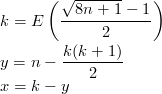## Numbering tiles

If we want a straightforward way to linearly index tiles without necessarily knowing the image size, we can use the following enumeration:

 0 1 3 6 10 15 21 2 4 7 11 16 22 29 5 8 12 17 23 30 38 9 13 18 24 31 39 … 14 19 25 32 40 … 20 26 33 41 … 27 34 42 …

One way to generate these values is using the Cantor polynomial:Efficiently inverting that polynomial is not trivial. Here is one way to do it:The following C code works OK for values up to 10,000 (higher values may cause overflows), which potentially allows for procedural terapixel images.

for(int y = 0; y < 10000; y++)
for(int x = 0; x < 10000; x++)
{
int n = (x + y) * (x + y + 1) / 2 + y;
int k = (sqrt((double)(n * 8 + 1)) - 1) / 2;
int y2 = n - k * (k + 1) / 2;
int x2 = k - y2;
if(x != x2 || y != y2)
printf("ERROR! %i %i -> %i -> %i %i\n", x, y, n, x2, y2);
}